# Explaining a fraud detection model with Cloud AI Platform

## 1. Overview

In this lab, you will use AI Platform Notebooks to build and train a model for identifying fraudulent transactions, and understand the model's predictions with the Explainable AI SDK. Fraud detection is a type of anomaly detection specific to financial services, and presents some interesting challenges for ML models: inherently imbalanced datasets and a need to explain a model's results.

## What you learn

You'll learn how to:

• Handle imbalanced datasets
• Build and evaluate a fraud detection model with tf.keras in AI Platform Notebooks
• Use the Explainable AI SDK from within the notebook to understand why the model classified transactions as fraudulent
• Deploy the model to AI Platform with explanations, and get predictions and explanations on the deployed model

The total cost to run this lab on Google Cloud is about \$1.

## 2. Why fraud detection?

Anomaly detection can be a good candidate for machine learning since it is often hard to write a series of rule-based statements to identify outliers in data. Fraud detection is a type of anomaly detection, and presents two interesting challenges when it comes to machine learning:

• Very imbalanced datasets: because anomalies are, well, anomalies, there are not many of them. ML works best when datasets are balanced, so things can get complicated when outliers make up less than 1% of your data.
• Need to explain results: if you're looking for fraudulent activity, chances are you'll want to know why a system flagged something as fraudulent rather than just take its word for it. Explainability tools can help with this.

## 3. Setup your environment

You'll need a Google Cloud Platform project with billing enabled to run this codelab. To create a project, follow the instructions here.

## Step 1: Enable the Cloud AI Platform Models API

Navigate to the AI Platform Models section of your Cloud Console and click Enable if it isn't already enabled.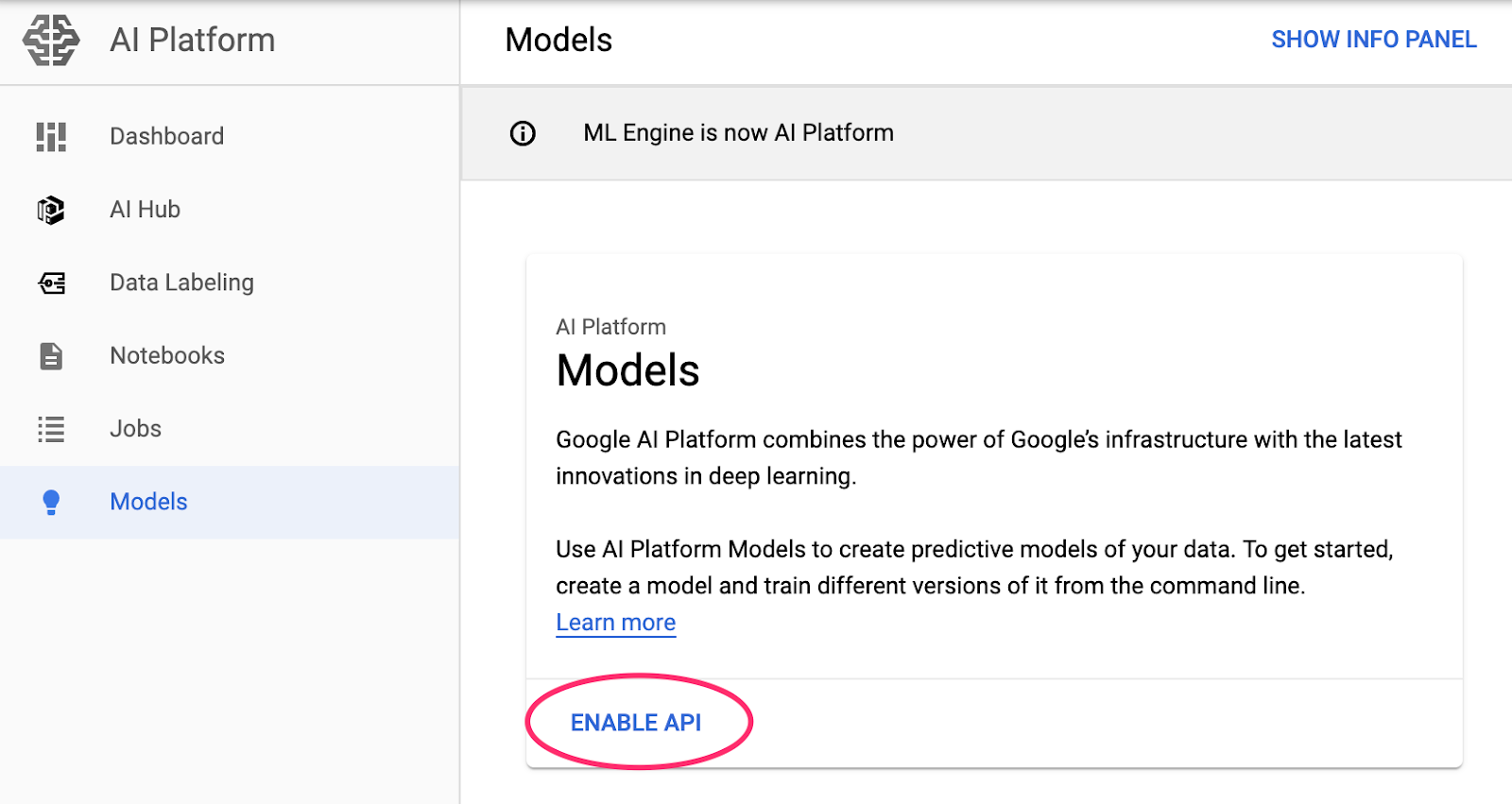## Step 2: Enable the Compute Engine API

Navigate to Compute Engine and select Enable if it isn't already enabled. You'll need this to create your notebook instance.

## Step 3: Create an AI Platform Notebooks instance

Navigate to AI Platform Notebooks section of your Cloud Console and click New Instance. Then select the TensorFlow Enterprise 2.1 instance type without GPUs: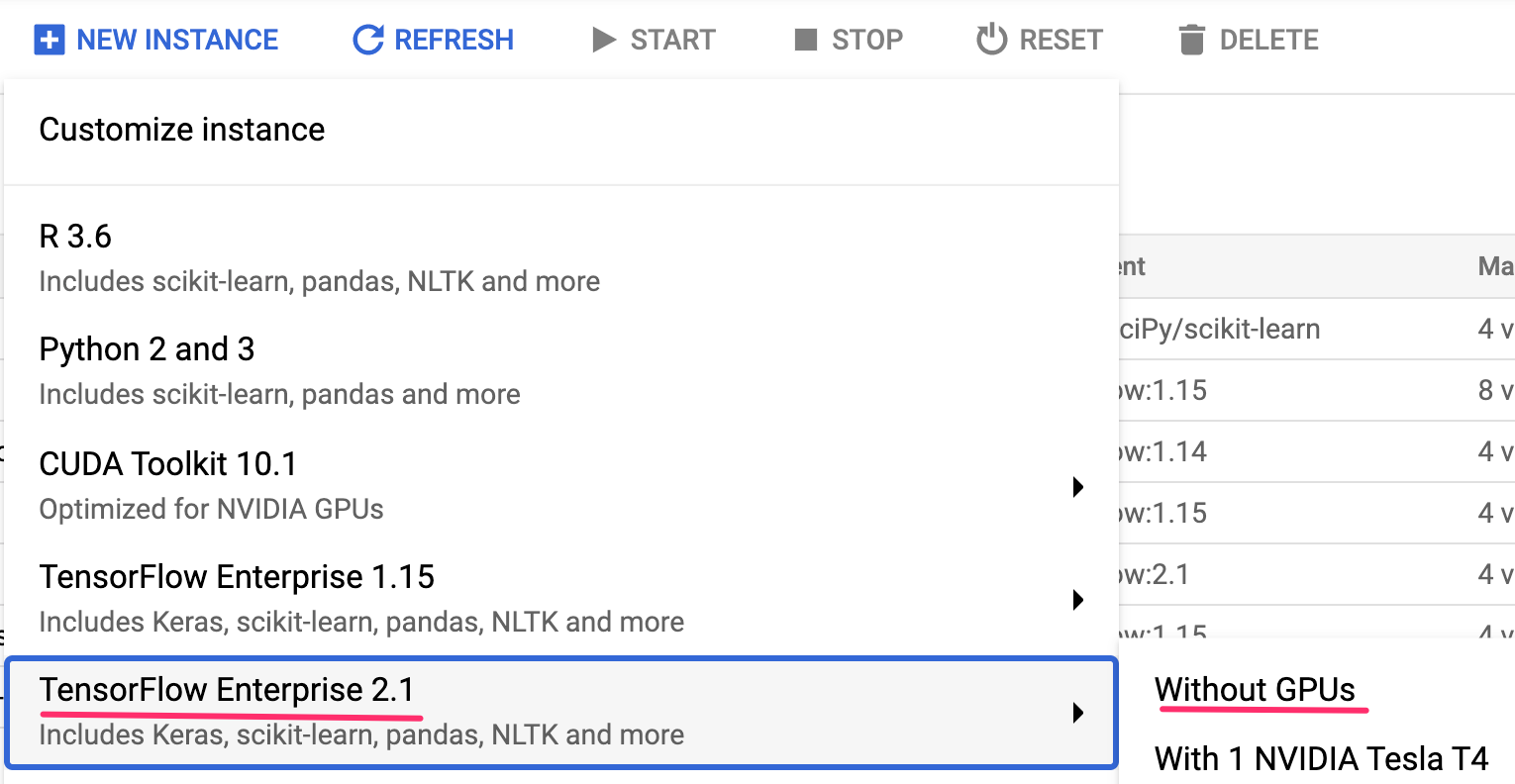Use the default options and then click Create. Once the instance has been created, select Open JupyterLab: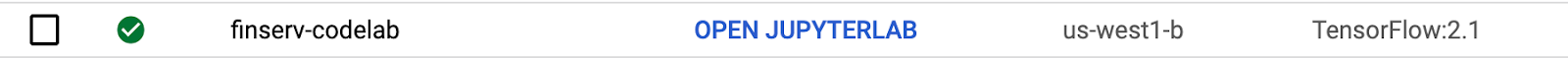When you open the instance, select Python 3 notebook from the launcher: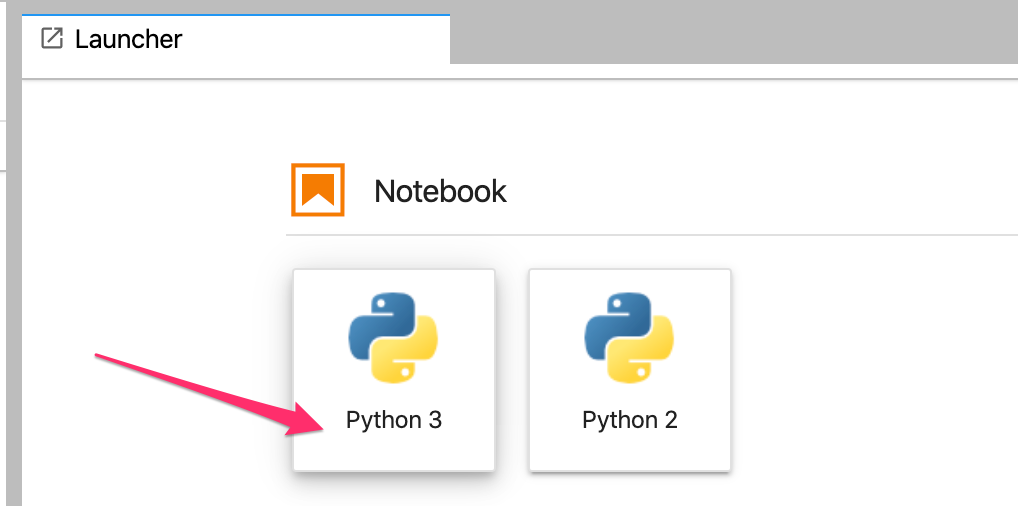## Step 4: Import Python packages

Create a new cell and import the libraries we'll be using in this codelab:

``````import itertools
import numpy as np
import pandas as pd
import tensorflow as tf
import json
import matplotlib as mpl
import matplotlib.pyplot as plt
import explainable_ai_sdk

from sklearn.utils import shuffle
from sklearn.metrics import confusion_matrix
from sklearn.preprocessing import StandardScaler
from tensorflow import keras
``````

We'll be using this synthetically generated dataset from Kaggle to train our model. The original dataset includes 6.3 million rows, 8k of which are fraudulent transactions - a mere 0.1% of the whole dataset!

## Step 1: Download the Kaggle dataset and read with Pandas

We've made the Kaggle dataset available for you in Google Cloud Storage. You can download it by running the following `gsutil` command in your Jupyter notebook:

``````!gsutil cp gs://financial_fraud_detection/fraud_data_kaggle.csv .
``````

Next, let's read the dataset as a Pandas DataFrame and preview it:

``````data = pd.read_csv('fraud_data_kaggle.csv')
data = data.drop(columns=['type'])
``````

You should see something like this in the preview: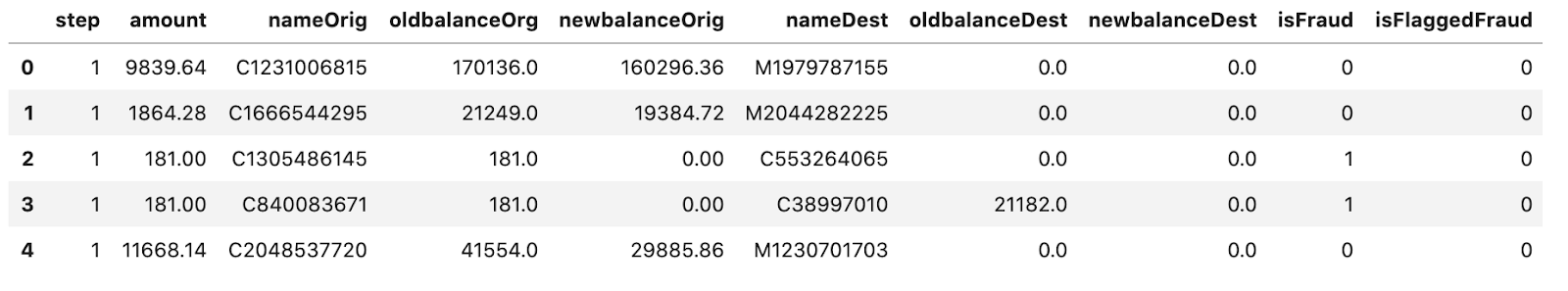## Step 2: Accounting for imbalanced data

As mentioned above, right now the dataset contains 99.9% non-fraudulent examples. If we train a model on the data as is, chances are the model will reach 99.9% accuracy by guessing every transaction is not a fraudulent one simply because 99.9% of the data is non fraudulent cases.

There are a few different approaches for dealing with imbalanced data. Here, we'll be using a technique called downsampling. Downsampling means using only a small percentage of the majority class in training. In this case, "non-fraud" is the majority class since it accounts for 99.9% of the data.

To downsample our dataset, we'll take all ~8k of the fraudulent examples and a random sample of ~31k of the non-fraud cases. This way the resulting dataset will have 25% fraud cases, compared to the .1% we had before.

First, split the data into two DataFrames, one for fraud and one for non-fraud (we'll make use of this later in the codelab when we deploy our model):

``````fraud = data[data['isFraud'] == 1]
not_fraud = data[data['isFraud'] == 0]
``````

Then, take a random sample of the non-fraud cases. We're using .005% since this will give us a 25/75 split of fraud / non-fraud transactions. With that, you can put the data back together and shuffle. To simplify things we'll also remove a few columns that we won't be using for training:

``````# Take a random sample of non fraud rows
not_fraud_sample = not_fraud.sample(random_state=2, frac=.005)

# Put it back together and shuffle
df = pd.concat([not_fraud_sample,fraud])
df = shuffle(df, random_state=2)

# Remove a few columns (isFraud is the label column we'll use, not isFlaggedFraud)
df = df.drop(columns=['nameOrig', 'nameDest', 'isFlaggedFraud'])

# Preview the updated dataset
``````

Now we've got a much more balanced dataset. However, if we notice our model converging around ~75% accuracy, there's a good chance it's guessing "non-fraud" in every case.

## Step 3: Split the data into train and test sets

The last thing to do before building our model is splitting our data. We'll use an 80/20 train-test split:

``````train_test_split = int(len(df) * .8)

train_set = df[:train_test_split]
test_set = df[train_test_split:]

train_labels = train_set.pop('isFraud')
test_labels = test_set.pop('isFraud')
``````

*E. A. Lopez-Rojas , A. Elmir, and S. Axelsson. "PaySim: A financial mobile money simulator for fraud detection". In: The 28th European Modeling and Simulation Symposium-EMSS, Larnaca, Cyprus. 2016

## 5. Build, train, and evaluate a tf.keras model

We'll be building using TensorFlow's tf.keras API. The model code in this section is built upon this tutorial from the TensorFlow docs. First we'll normalize the data, and then we'll build and train our model, using the `class_weight` parameter to account for the remaining data imbalance.

## Step 1: Normalize the data

When training a model on numerical data, it's important to normalize the data, especially if each column falls in a different range. This can help prevent loss from exploding during training. We can normalize our data with the following:

``````scaler = StandardScaler()
train_set = scaler.fit_transform(train_set) # Only normalize on the train set
test_set = scaler.transform(test_set)

# clip() ensures all values fall within the range [-5,5]
# useful if any outliers remain after normalizing
train_set = np.clip(train_set, -5, 5)
test_set = np.clip(test_set, -5, 5)
``````

Then, let's preview our normalized data:

``````train_set
``````

## Step 2: Determine class weights

When downsampling the data, we still wanted to keep a subset of the non-fraudulent transactions so we didn't lose information on those transactions, which is why we didn't make the data perfectly balanced. Because the dataset is still imbalanced and we care most about correctly identifying fraudulent transactions, we want our model to give more weight to fraudulent examples in our dataset.

The Keras `class_weight` parameter lets us specify exactly how much weight we want to give examples from each class, based on how often they occur in the dataset:

``````weight_for_non_fraud = 1.0 / df['isFraud'].value_counts()
weight_for_fraud = 1.0 / df['isFraud'].value_counts()

class_weight = {0: weight_for_non_fraud, 1: weight_for_fraud}
``````

We'll use this variable when we train our model in the next step.

## Step 3: Train and evaluate the model

We'll build our model using the Keras Sequential Model API, which lets us define our model as a stack of layers. There are a number of metrics we'll track as we're training, which will help us understand how our model is performing on each class in our dataset.

``````METRICS = [
keras.metrics.TruePositives(name='tp'),
keras.metrics.FalsePositives(name='fp'),
keras.metrics.TrueNegatives(name='tn'),
keras.metrics.FalseNegatives(name='fn'),
keras.metrics.BinaryAccuracy(name='accuracy'),
keras.metrics.Precision(name='precision'),
keras.metrics.Recall(name='recall'),
keras.metrics.AUC(name='auc'),
]

def make_model(metrics = METRICS):
model = keras.Sequential([
keras.layers.Dense(
16, activation='relu',
input_shape=(train_set.shape[-1],)),
keras.layers.Dropout(0.5),
keras.layers.Dense(1, activation='sigmoid'),
])

model.compile(
loss=keras.losses.BinaryCrossentropy(),
metrics=metrics)

return model
``````

Then, we'll define a few global variables for use during training along with some early stopping parameters.

``````EPOCHS = 100
BATCH_SIZE = 512

early_stopping = tf.keras.callbacks.EarlyStopping(
monitor='val_auc',
verbose=1,
patience=10,
mode='max',
restore_best_weights=True)
``````

Finally, we'll call the function we defined above to make our model:

``````model = make_model()
model.summary()
``````

We can train our model with the `fit()` method, passing in parameters defined above:

``````results = model.fit(
train_set,
train_labels,
batch_size=BATCH_SIZE,
epochs=EPOCHS,
callbacks = [early_stopping],
validation_data=(test_set, test_labels),
class_weight=class_weight)
``````

Training will take a few minutes to run.

## Step 4: Visualize model metrics

Now that we have a trained model, let's see how our model performed by plotting various metrics throughout our training epochs:

``````mpl.rcParams['figure.figsize'] = (12, 10)
colors = plt.rcParams['axes.prop_cycle'].by_key()['color']

def plot_metrics(history):
metrics =  ['loss', 'auc', 'precision', 'recall']
for n, metric in enumerate(metrics):
name = metric.replace("_"," ").capitalize()
plt.subplot(2,2,n+1)
plt.plot(history.epoch,  history.history[metric], color=colors, label='Train')
plt.plot(history.epoch, history.history['val_'+metric],
color=colors, linestyle="--", label='Val')
plt.xlabel('Epoch')
plt.ylabel(name)
if metric == 'loss':
plt.ylim([0, plt.ylim()])
elif metric == 'auc':
plt.ylim([0.8,1])
else:
plt.ylim([0,1])

plt.legend()

plot_metrics(results)
``````

Your graphs should look similar to the following (but won't be exactly the same):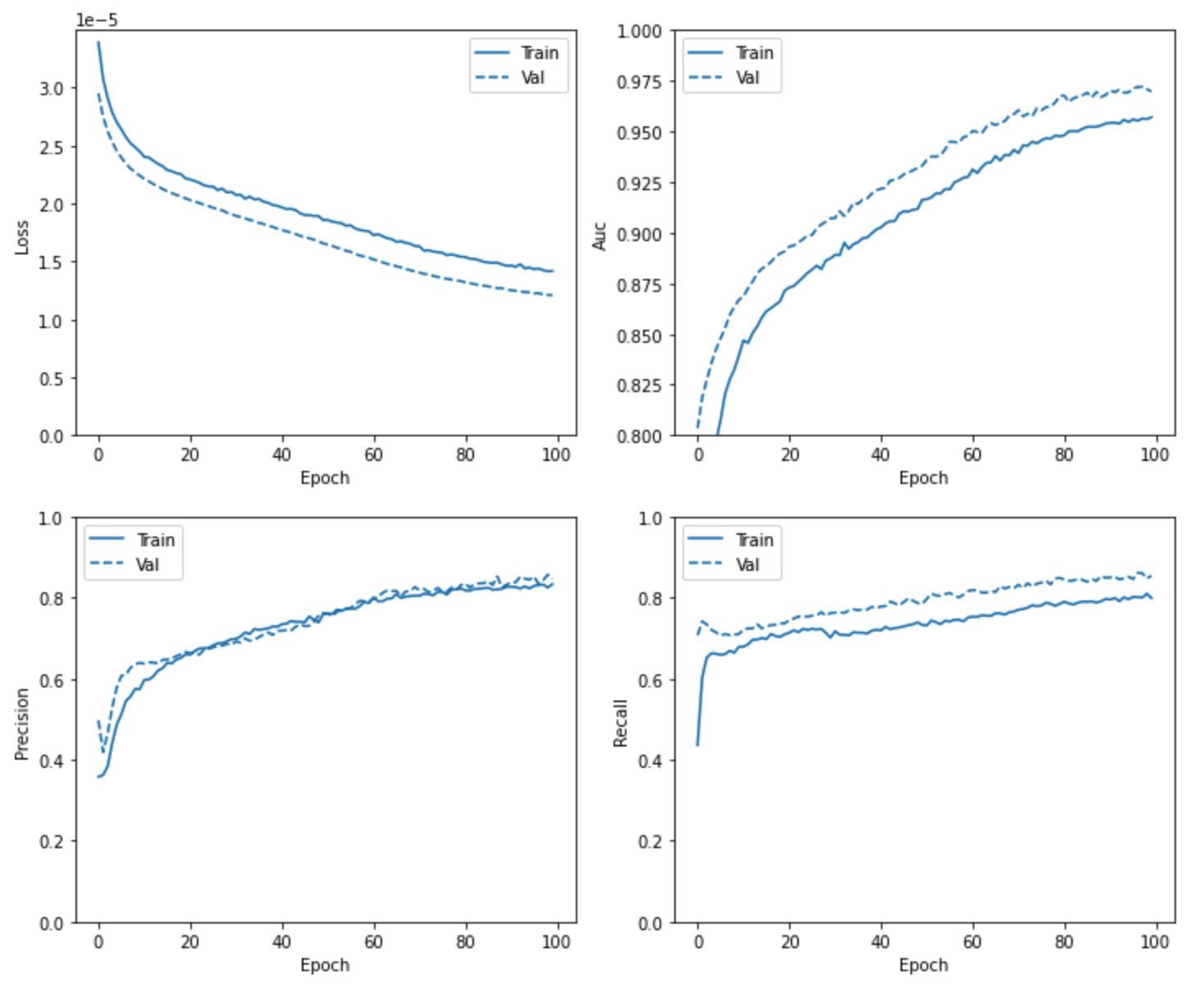## Step 5: Print a confusion matrix

A confusion matrix is a nice way to visualize how our model performed across the test dataset. For each class, it will show us the percentage of test examples that our model predicted correctly and incorrectly. Scikit Learn has some utilities for creating and plotting confusion matrices, which we'll use here.

At the beginning of our notebook we imported the `confusion_matrix` utility. To use it, we'll first create a list of our model's predictions. Here we'll round the values returned from our model so that this lists matches our list of ground truth labels:

``````predicted = model.predict(test_set)

y_pred = []

for i in predicted.tolist():
y_pred.append(int(round(i)))
``````

Now we're ready to feed this into the `confusion_matrix` method, along with our ground truth labels:

``````cm = confusion_matrix(test_labels.values, y_pred)
print(cm)
``````

This shows us the absolute numbers of our model's correct and incorrect predictions on our test set. The number on the top left shows how many examples from our test set our model correctly predicted as non-fraudulent. The number on the bottom right shows how many it correctly predicted as fraudulent (we care most about this number). You can see that it predicted the majority of samples correctly for each class.

To make this easier to visualize, we've adapted the `plot_confusion_matrix` function from the Scikit Learn docs. Define that function here:

``````def plot_confusion_matrix(cm, classes,
normalize=False,
title='Confusion matrix',
cmap=plt.cm.Blues):
"""
This function prints and plots the confusion matrix.
Normalization can be applied by setting `normalize=True`.
"""
plt.imshow(cm, interpolation='nearest', cmap=cmap)
plt.title(title)
plt.colorbar()
tick_marks = np.arange(len(classes))
plt.xticks(tick_marks, classes, rotation=45)
plt.yticks(tick_marks, classes)

if normalize:
cm = np.round(cm.astype('float') / cm.sum(axis=1)[:, np.newaxis], 3)

thresh = cm.max() / 2.
for i, j in itertools.product(range(cm.shape), range(cm.shape)):
plt.text(j, i, cm[i, j],
horizontalalignment="center",
color="white" if cm[i, j] > thresh else "black")

plt.tight_layout()
plt.ylabel('True label')
plt.xlabel('Predicted label')
``````

And create the plot by passing it the data from our model. We're setting `normalize` to `True` here so that the confusion matrix displays the number of correct and incorrect predictions as percentages:

``````classes = ['not fraud', 'fraud']
plot_confusion_matrix(cm, classes, normalize=True)
``````

You should see something like this (exact numbers will vary):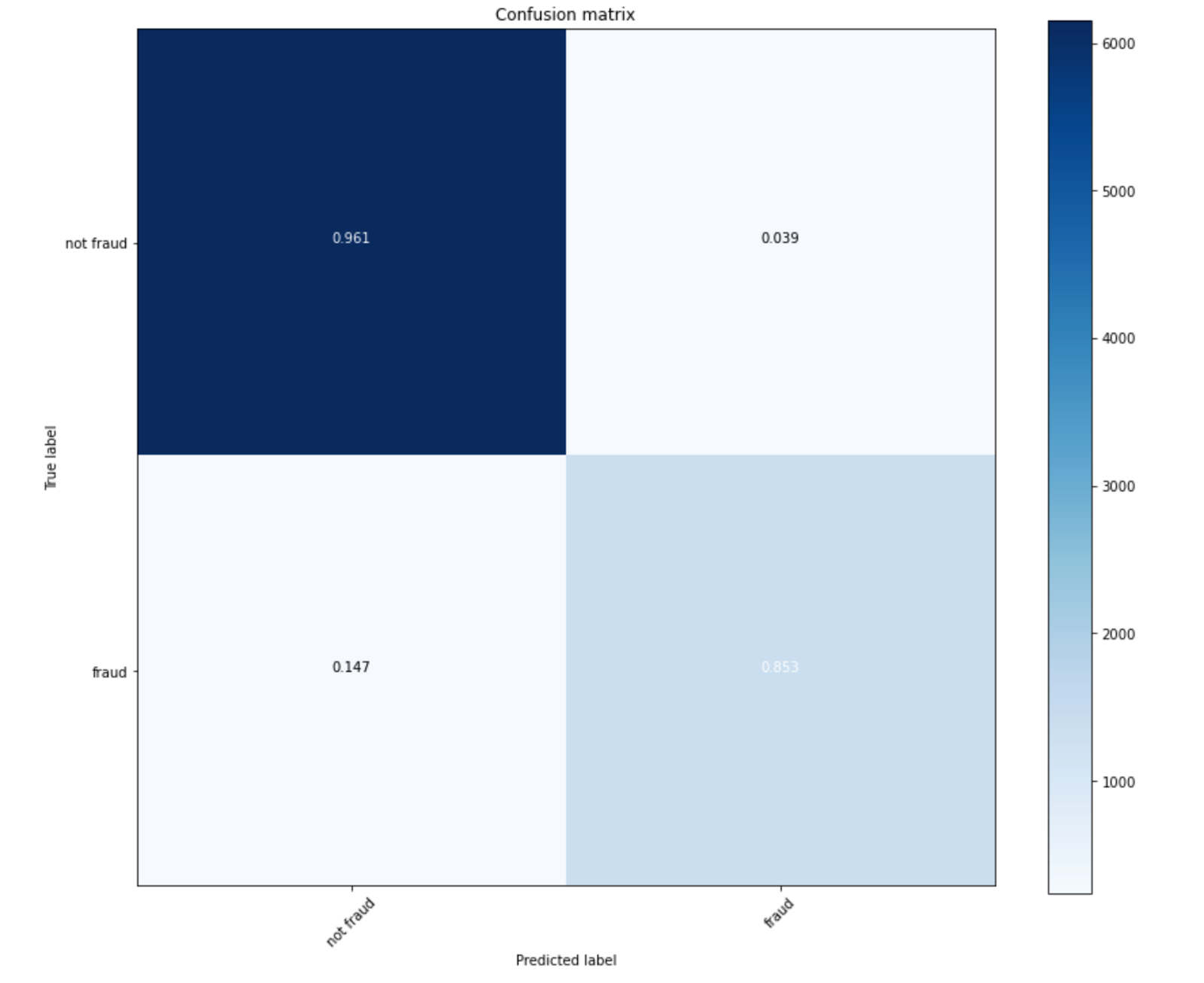Here we can see that our model predicted around 85% of the 1,594 fraudulent transactions from our test set correctly. Note that the focus in this lab is not on model quality – if you're deploying a fraud detection model in production you'd likely want higher than 85% accuracy on the fraud class. The goal of this lab is to introduce you to the tooling around explaining models trained on imbalanced datasets.

Next, we'll use the Explainable AI SDK to understand which features our model is relying on to make these predictions.

## 6. Using the Explainable AI SDK

The Explainable AI SDK provides utility methods for getting explanations on your model. It comes pre-installed in Tensorflow AI Platform Notebook instances – note that we imported it in our notebook at the beginning of our lab. With the SDK, we can get feature attributions from our model within the notebook instance, which means we don't need to deploy our model to the cloud to use it.

In this section, we'll export the model we just trained as a Tensorflow SavedModel, and then point the SDK at our saved model assets to get explanations.

## Step 1: Export trained model

First, let's save our model into a directory in our notebook instance:

``````model_dir = 'fraud_model'
tf.saved_model.save(model, model_dir)
``````

If you refresh the folder view in the left sidebar of your notebook, you should see a new directory called `fraud_model/` created.

## Step 2: Get explanation metadata with the SDK

Next, we'll point the Explainable AI SDK at that directory. Doing this will generate metadata necessary for getting model explanations. The `get_metadata()` method shows metadata the SDK infers from your model, like input names:

``````model_builder = SavedModelMetadataBuilder(model_dir)
``````

Explainability helps us answer the question: "Why did our model think this was fraud?"

## Step 3: Specifying our model's baseline

For tabular data, the Explainable AI service works by returning attribution values for each feature. These values indicate how much a particular feature affected the prediction. Let's say the amount of a particular transaction caused our model to increase its predicted fraud probability by 0.2%. You might be thinking "0.2% relative to what??". That brings us to the concept of a baseline.

The baseline for our model is essentially what it's comparing against. We select the baseline value for each feature in our model, and the baseline prediction consequently becomes the value our model predicts when the features are set at the baseline.

Choosing a baseline depends on the prediction task you're solving. For numerical features, it's common to use the median value of each feature in your dataset as the baseline. In the case of fraud detection, however, this isn't exactly what we want. We care most about explaining the cases when our model labels a transaction as fraudulent. That means the baseline case we want to compare against is non-fraudulent transactions.

To account for this, we'll use the median values of the non-fraudulent transactions in our dataset as the baseline. We can get the median by using the `not_fraud_sample` DataFrame we extracted above, and scaling it to match our model's expected inputs:

``````not_fraud_sample = not_fraud_sample.drop(columns=['nameOrig', 'nameDest', 'isFlaggedFraud', 'isFraud'])

baseline = scaler.transform(not_fraud_sample.values)
baseline = np.clip(baseline, -5, 5)
baseline_values = np.median(baseline, axis=0)
``````

Note that we don't need to specify a baseline. If we don't, the SDK will use 0 as a baseline for each input value our model is expecting. In our fraud detection use case, it makes sense to specify a baseline, which we'll do below:

``````input_name = list(metadata['inputs'])
``````

Running the `save_metadata()` method above created a file in our model's directory called `explanation_metadata.json`. In your notebook, navigate to the fraud_model/ directory to confirm that file was created. This contains metadata that the SDK will use to generate feature attributions.

## Step 4: Getting model explanations

We're now ready to get feature attributions on individual examples. To do that, we'll first create a local reference to our model using the SDK:

``````local_model = explainable_ai_sdk.load_model_from_local_path(
model_dir,
explainable_ai_sdk.SampledShapleyConfig()
)
``````

Next, let's get predictions and explanations on our model from an example transaction that should be classified as fraudulent:

``````fraud_example = [0.722,0.139,-0.114,-0.258,-0.271,-0.305]
response = local_model.explain([{input_name: fraud_example}])
``````

Running this should create a visualization that looks like the following: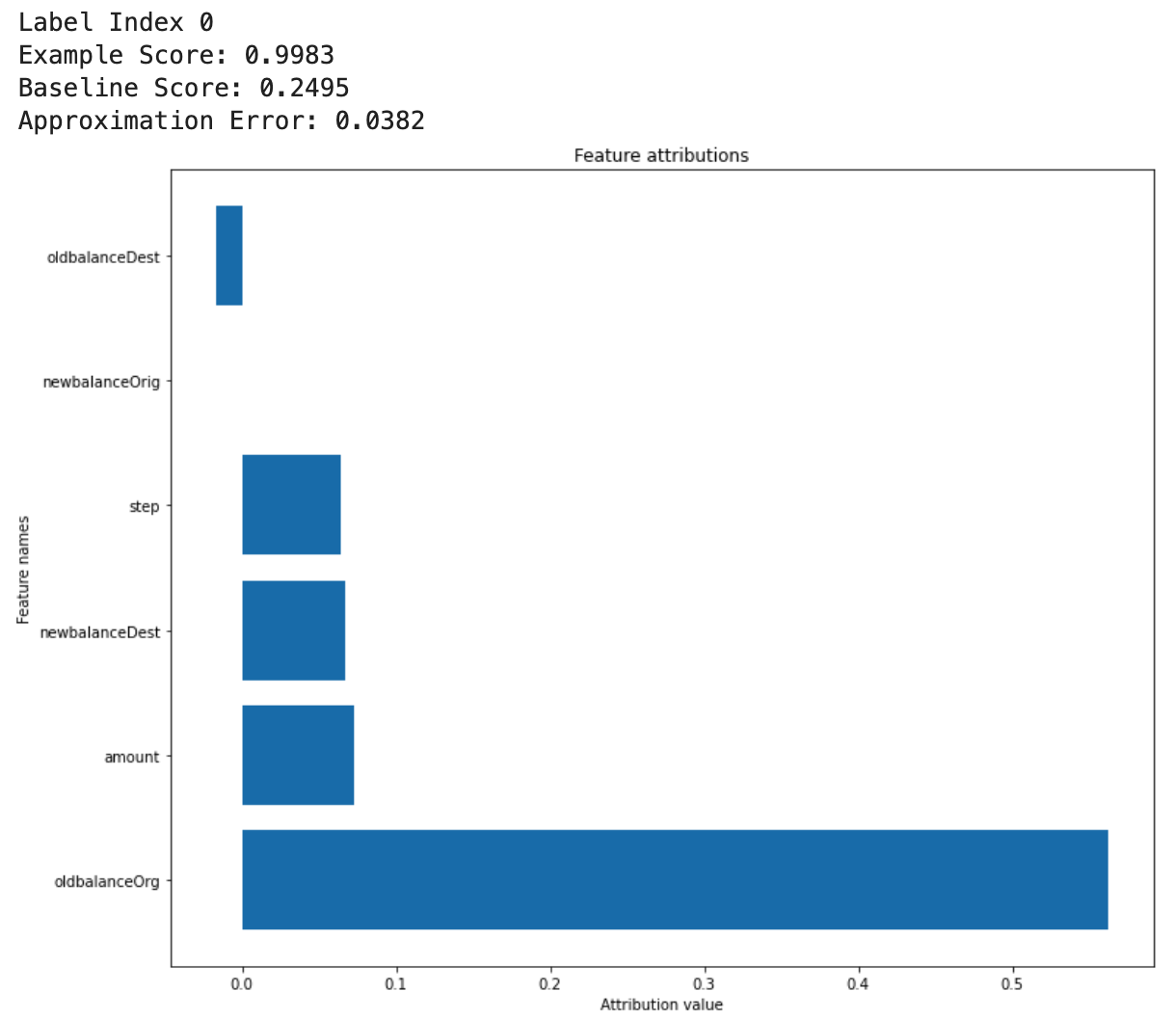In this example, the account's initial balance before the transaction took place was the biggest indicator of fraud, pushing our model's prediction up from the baseline more than 0.5. The transaction's amount, resulting balance at the destination account, and step were the next biggest indicators. In the dataset, the "step" represents a unit of time (1 step is 1 hour). Attribution values can also be negative.

The "approximation error" that is printed above the visualizations lets you know how much you can trust the explanation. Generally, error over 5% means you may not be able to rely on the feature attributions. Remember that your explanations are only as good as the training data and model you used. Improving your training data, model, or trying a different model baseline can decrease the approximation error.

You may also be able to decrease this error by increasing the number of steps used in your explanation method. You can change this with the SDK by adding a `path_count` parameter to your explanation config (the default is 10 if you don't specify):

``````local_model = explainable_ai_sdk.load_model_from_local_path(
model_dir,
explainable_ai_sdk.SampledShapleyConfig(path_count=20)
)
``````

There is lots more you can do with Explainable AI on this model. Some ideas include:

• Sending many examples to our model and averaging the attribution values to see if certain features are more important overall. We could use this to improve our model, and potentially remove features that aren't important
• Finding false positives that our model flags as fraud but are non-fraudulent transactions, and examining their attribution values
• Use a different baseline and see how this impacts the attribution values

🎉 Congratulations! 🎉

You've learned how to account for imbalanced data, train a TensorFlow model to detect fraudulent transactions, and use the Explainable AI SDK to see which features your model is relying on most to make individual predictions. You can stop here if you'd like. Using the SDK within a notebook is meant to simplify your model development process by giving you access to explanations before you deploy a model. Chances are once you've built a model you're happy with, you'd like to deploy it to get predictions at scale. If that sounds like you, continue to the optional next step. If you're done, skip to the Cleanup step.

## 7. Optional: Deploy the model to AI Platform Prediction

In this step, you'll learn how to deploy your model to AI Platform Prediction.

## Step 1: Copy your saved model directory to a Cloud Storage Bucket.

With the SDK steps we ran previously, you have everything you need to deploy your model to AI Platform. To prepare for deployment, you'll need to put your SavedModel assets and explanation metadata in a Cloud Storage Bucket that the Explainable AI service can read.

To do that, we'll define some environment variables. Fill in the values below with the name of your Google Cloud project and the name of the bucket you'd like to create (must be globally unique).

``````# Update these to your own GCP project and model
GCP_PROJECT = 'your-gcp-project'
MODEL_BUCKET = 'gs://storage_bucket_name'
``````

Now we're ready to create a storage bucket to store our exported TensorFlow model assets. We'll point AI Platform at this bucket when we deploy the model.

Run this `gsutil` command from within your notebook to create a bucket:

``````!gsutil mb -l 'us-central1' \$MODEL_BUCKET
``````

Then, copy your local model directory into that bucket:

``````!gsutil -m cp -r ./\$model_dir/* \$MODEL_BUCKET/explanations
``````

## Step 2: Deploy the model

Next, we'll define some variables we'll use in our deployment commands:

``````MODEL = 'fraud_detection'
VERSION = 'v1'
model_path = MODEL_BUCKET + '/explanations'
``````

We can create the model with the following gcloud command:

``````!gcloud ai-platform models create \$MODEL --region=us-central1
``````

Now we're ready to deploy our first version of this model with gcloud. The version will take ~5-10 minutes to deploy:

``````!gcloud beta ai-platform versions create \$VERSION \
--model \$MODEL \
--origin \$model_path \
--runtime-version 2.1 \
--framework TENSORFLOW \
--python-version 3.7 \
--machine-type n1-standard-4 \
--explanation-method 'sampled-shapley' \
--num-paths 10 \
--region=us-central1
``````

In the `origin` flag, we pass in the Cloud Storage location of our saved model and metadata file. Explainable AI currently has two different explanation methods available for tabular models. Here we're using Sampled Shapley. The `num-paths` parameter indicates the number of paths sampled for each input feature. Generally, the more complex the model, the more approximation steps are needed to reach reasonable convergence.

To confirm your model deployed correctly, run the following gcloud command:

``````!gcloud ai-platform versions describe \$VERSION --model \$MODEL --region=us-central1
``````

The state should be `READY`.

## Step 3: Getting predictions and explanations on the deployed model

For the purposes of explainability, we care most about explaining the cases where our model predicts fraud. We'll send 5 test examples to our model that are all fraudulent transactions.

We'll use the Google Cloud CLI to get predictions. Run the following code to get the indices of all of the fraud examples from our test set:

``````fraud_indices = []

for i,val in enumerate(test_labels):
if val == 1:
fraud_indices.append(i)
``````

Next we'll save 5 examples in the format our model is expecting, and write them to a file:

``````num_test_examples = 5

instances = []
for i in range(num_test_examples):
ex = test_set[fraud_indices[i]]
instances.append({input_name: ex.tolist()})

with open('prediction.json', 'a') as outputfile:
json.dump({"instances": instances}, outputfile)
``````

We can send these five examples to our model using gcloud:

``````!gcloud beta ai-platform explain \
--model=\$MODEL \
--version=\$VERSION \
--region='us-central1' \
--json-request=prediction.json
``````

In the response JSON, you'll see the attribution values for each feature across these examples. The `example_score` key for each example includes the model's prediction - in this case the percentage likelihood that a particular transaction is fraudulent.

## 8. Cleanup

If you'd like to continue using this notebook, it is recommended that you turn it off when not in use. From the Notebooks UI in your Cloud Console, select the notebook and then select Stop: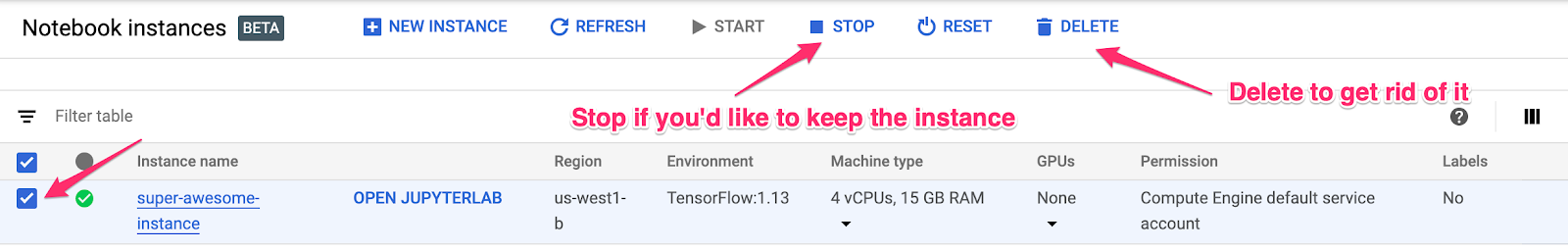If you'd like to delete all resources you've created in this lab, simply delete the notebook instance instead of stopping it.

Using the Navigation menu in your Cloud Console, browse to Storage and delete both buckets you created to store your model assets.

[{ "type": "thumb-down", "id": "missingTheInformationINeed", "label":"Missing the information I need" },{ "type": "thumb-down", "id": "tooComplicatedTooManySteps", "label":"Too complicated / too many steps" },{ "type": "thumb-down", "id": "outOfDate", "label":"Out of date" },{ "type": "thumb-down", "id": "samplesCodeIssue", "label":"Samples / code issue" },{ "type": "thumb-down", "id": "otherDown", "label":"Other" }]
[{ "type": "thumb-up", "id": "easyToUnderstand", "label":"Easy to understand" },{ "type": "thumb-up", "id": "solvedMyProblem", "label":"Solved my problem" },{ "type": "thumb-up", "id": "otherUp", "label":"Other" }]